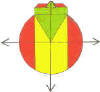DMD   EDU-HOME

DON'T LET  THIS CALCULATION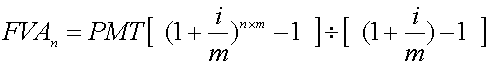OR THIS FORMULA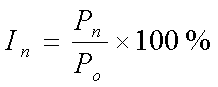SCARE  YOU!!

Central to any business enterprise is the generation of profit, that is a monetary return on an input of capital, services and/or the provision of products.   Concepts such as capital, assets, liabilities, solvency, liquidity, dividends are fundamental in the understanding of the functioning of a business.

It is not the objective of this site to provide any information on mentioned concepts.  What we intend doing is to extract mathematics-related problems from suitable resource material.

Knowledge of basic mathematics is essential to a more comprehensive understanding of the business essence of profit-making.

Possessing a business calculator is not sufficient; knowing how to use it effectively is helpful, but may still require knowledge of mathematical basics such as the ability to understand and work with brackets.

Important areas of basic mathematics are:

·              Number Concept;

·              Basic mathematics operations of addition, subtraction, division and multiplication;

·              Fractions, Decimal Factions and Percentages (See Below);

·              Ratio and Proportion (See Below);

·              Interest, essentially Compound Interest;

·             Calculator Skills;

·

A:  PROBLEMS ON PERCENTAGES with solutions

1. If the VAT (VALUE-ADDED TAX) on an article amounts to

(a)   R14;

(b)   R12.28

what is the price of the article, excluding VAT ( Remember: In   South Africa, VAT = 14% of amount).

SOLUTION: (a)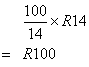(b)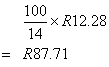2. Express ¾ % as a

(a)   ordinary (or vulgar) fraction;

(b)   decimal fraction.

SOLUTION: (a)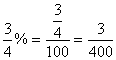(b)    3÷400= 0.0075

3. If the Index for all shares ended on 11 October 2002 by 279.59 points higher on  9398.87 , verify that this increase represents an increase of 3.07%.

SOLUTION:     PRICE INCREASE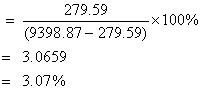4. If the gold price ended 0.6% higher on 316.65 dollars, what was the gold price originally before the increase?

SOLUTION:     ORIGINAL GOLD PRICE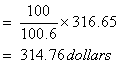B:  PROBLEMS ON RATIO AND PROPORTIONS with solutions

1. On Friday 27 July 2007,  7.10 South African (SA) rands or ZAR bought 1 US dollar while 14.4 SA rands (ZAR) bought 1 British pound. If the rand/dollar rate remained unchanged from the previous day, while the rand/British pound rate dropped by 0.36%, what should the dollar/British pound rate the previous day, 26 July 2007 have been? (The rate for the US\$ and British pound was 2.0488 USD per one British pound on that day. What conclusions do you draw?)

SOLUTION:

Present US dollar/pound rate =dollars for one British pound.

Previous day’s dollar/British pound rate =dollar for one British pound.

Should you wish to comment, send them to:

PROBLEMS EXTRACTED FROM:

THE CAPE ARGUS & DIE BURGER: 5 October 2002

1. In September 2001,  315 tractors were sold.  During September 2002  46% more tractors were sold than in the corresponding previous year month.   How many tractors were sold in September 2002?

2. Check the correctness of the following table:

SALES OF FARM MACHINERY

 Sept % Change Sept % Change 2000 2001 2000 2001 Tractors 461 315 3 079 2055 49.8 "Stropers" Harvesters 16 7 128.6 139 60 131.7 "Balers" 14 7 100 137 130 5.4

3. Draw a pie-chart diagram to represent the share of each of the following South African language groups in the marketing of products:

Afrikaans Speakers:            36.3%

English         "                      35%

Sotho/Shona     "                  6.1%

Tsonga/Venda    "                 0.9%

Zulu/Xhosa      "                   7.9%

Others                                    ?

4. The solvency ratio, expressed as a percentage, of the Pro Sano Medical Aid Group in 2001 is 39.64%.  Use the following information to derive the formula to calculate the solvency ratio, and hence check the validity of the solvency ratio of 39.64% .

Average Member Contribition             R 430

Average Member Benefit                     R 373

Current Assets                      R 386 077 103

Current Liabilties                 R 102 517 788

Reserves                               R 258 277 000

Membership Numbers                      126 593

Legal reserve requiremenent or

Solvency level of contributions              13.5%

5. If your monthly medical aid fund contribution of R2 150 is increased by 12.4%, how much more will you now pay per month?

6. How long will it take you to repay a bank loan of R7 000 at R665 per month at a rate of 16.25% p.a.

7. You wish to retire at age 50.   How much do you need to save each month if you start saving a fixed amount monthly at age 30, and assuming a 15% investment return?

8. Use the following formula to determine  how much money R will be sufficient for retirement:

R = 100/(r - i) × A

where r is the (expected) annual rate of investment;

i  is the annual rate of inflation;

and       A is the (expected) annual expenses.

(i)  Determine R if r = 12.25   i = 7.5 and A = R125 700.

(ii) Determine r if R = R3.5 million, i = 8 and A = R114 000.

9.  How long will it take R250 to double itself if invested at 7.8% compound interest, interest added monthly on the minimum balance?

TERM TEST 1                                                         March 2008

Question 1

A group of 18 students decide to go on a weekend outing of two days. Their expenses will be based on 320 kilometres of traveling, and the following:

·        R550  daily rental for a 12 seater mini-bus plus R1.50 per kilometre;

·        R7.04 per litre of petrol; (Use: 1 litre of petrol covers 10 km)

·        R239 per day each to hire 4 bungalows.

·        R300 daily rental plus R1.25 per kilometer for a double cab.

The students all agree  to pay as follows:

·                    The total expense is divided equally amongst themselves;

·                    The drivers who each pay 9.8% less than their mates;

·                    Those who withdraw each have to pay R100 penalty fee.

Calculate the total amount each one has to pay, if two of them withdraw and decide not to join the week-end outing.

SOLUTION:

MINI-BUS           PETROL           BUNGALOWS       DOUBLE CAB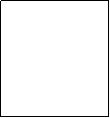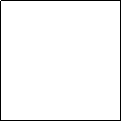2×550              2×32×R7.04            4×2×R239                  2×300

+                                                                                      +

1.5×320                                                                          1.25×320

TOTAL COSTR1 580 + R450.56 + R1 912 + R1 000

=R4 942.56

TWO DON’T GOR4 942.56 –R200

16 HAVE TO PAY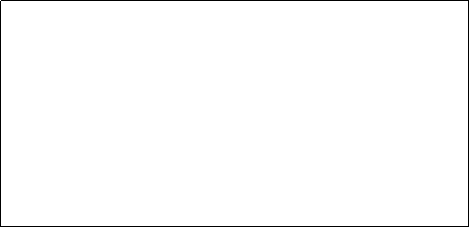LET x be the price 14 pay, then 2 will pay 2×x×0.902

14×x  + 2×x ×0. 902 = 4742.56

15. 804   = 4742.56

x    = 300 . 09

Therefore the drivers pay ……..

Question 6

Four amounts in South African currency are:      2.05 million rands,  17 800 999 cents,  20 168.09 rands and 12 125.5 cents.   If 62% of a fifth amount equals  0.056 million cents,  what is twice the total of these five amount plus another ½ million rands altogether?

CLASS TEST 5                                                                                                                                  October 2007

QUESTION 1

Solve for the unknown (and perform a check of your answer for BOTH 1.1 and 1.2)

1.1       –0.3(0.3P+0.5P) + 0.8P = 0.5P –2.4          3.2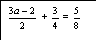(6)

CHECK:                                                   CHECK:

1.3                                                                                                                   (2)

QUESTION 2

Solve simultaneously for p and q:(4)

QUESTION 3

Ntandi, who share profits with Neil in the ratio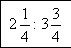, received R 215 000 of the profit.

(a)      Calculate the half of Neil’s share;

(b)           What is 150% of the total profit?                                                                                                                                            (3)

QUESTION 4

Two adults and three children attended a South African musical in Cape Town .  The total cost of entrance was R160, but they received a discount of  25% on all the tickets. Each adult paid R10 more than each child.  Find the amount which each adult and each child paid for their tickets.                                                                                                                        (6)

QUESTION 5

A rectangle is a cm long and b cm wide. The perimeter of the rectangle is 48 cm and the length is 5cm more than the width. Find the length of the rectangle                  (4)

QUESTION 6

It costs a total of R61,20 to purchase 10 lipsticks and 12 combs in a store. If the store raises the price of lipsticks by 20% and the price of combs by 10%, the total price of these items becomes R70. Find the original price of

(a)                two lipsticks;

(b)               one comb;

(c)        five lipsticks and seven combs.                                                             (5)

QUESTION  7

The supply of units of a certain product per month is given by the function S(x) =

y =  15.75x.  The demand for these units per month is given by the function D(x) =

y =  –9x + 55,  where   y  =  price of the product in R1 000’s;  x  = number of the product in 100’s,  supplied or sold in a month.

Determine the point of equilibrium algebraically.                                                 (5)

TERM TEST

2 DURATION: 2 hours                                                                      October 2007

TOTAL: 65 marks

QUESTION 1 (4 marks)

Given the expression: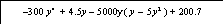write down / give

(a) the simplified expression  in  descending powers of y;

(b) the number of terms in the above unsimplified expression;

(c) the degree of the expression in y;

(d) the coefficient of  y²  in the simplified expression.

(4)

QUESTION 2 (8 marks)

(a) Simplify:      –8x – 13(x –4y)  + 5x –17y                                                                (1)

(b) If P(x)=-300x–40+500, R(x) =70.5x and C(x) = -x+20400                                                determine:

1.   R(x) – C(x)

2.   P(x)  + C(x)                                                                                               (2)

(c) Subtract twice the sum ofandfrom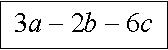.                                                                              (3)

(d) Simplify:

(i)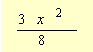of  (-80x – 248xy)                                                                  (1)

(ii)(1)

QUESTION 3 (4 marks)

Calculate the value of the expressions,  if:

a = –0.5  ;  b = 3   and   c = -5

(a)     -a + b - 3c

(b)  a³b – (a+c)(a–c)                                       (4)

QUESTION 4 (8 marks)

Simplify COMPLETELY:

(a)                  (2ax – 5y)(2ax + 5y)

(b)

(c)

(d)

(8)

QUESTION 5 (6 marks)

Simplify:

(a)

(b)                                                                                           (6)

QUESTION 6 (6 marks)

(a) Write  as a decimal fraction.

(b) Write      as a percentage.

(c)  Write 0.009609 in the form  where   0<A<10 and n is an integer.

(d)  Write 1 709.010 08  as a decimal number.

(6)

QUESTION 7 (6 marks)

Solve for the unknown (and perform a check of your answer for 7(a) ONLY):

(a)   –0.2(3c-3) + 0.8c = 0.5c -2.4         (b)                                                             (4)

CHECK:

(c)                                                                                                             (2)

QUESTION 8 (3 marks)

Determine the unknowns which satisfy both equations simultaneously:                  and                                   (3)

QUESTION 9 (3 marks)

Divide R16 into 10c pieces and 20c pieces such that there are twice times as many 10c pieces as 20c pieces.

(3)

QUESTION 10 (4 marks)

R60 and R80 tickets were sold for a certain entertainment show. The total income for the show was R22 000.  If there we altogether 400 people who attended the show, of whom 9.25% did not pay for their tickets (since the received them free as patrons) how many of each ticket price were sold?                                                                                                                          (4)

QUESTION 11 (4 marks)

The perimeter of a rectangular field is 112 metres.  The length is two hundred and fifty percent (250%) of the breadth.  Find:

(a)    The length and breadth of the field.

(3)

(b)   The area of the field.                                                                                         (1)

QUESTION 12 (5 marks)

It costs a total of R61.20 to purchase 5 lipsticks and 6 combs in a store. If the store raises the price of lipsticks by 40% and the price of combs by 20%, the total price of these items becomes R70.76. Find the original price, to the nearest cent, of

(a)                two lipsticks;

(b)               one comb;

(c)    five lipsticks and seven combs

(5)

QUESTION  13 (4 marks)

The supply of units of a certain product per month is given by the function S(x) =

y =  12.25x.  The demand for these units per month is given by the function D(x) =

y = –9.5x + 565.5     where   y  =  price of the product in R1 000’s;  x  = number of the product in 100’s,  supplied or sold in a month.

Determine the point of equilibrium algebraically.( That is, determine the values of x and y for which supply equals demand.)                                                                                    (4)

PROBLEMS : 30 APRIL 2008

MEASUREMENT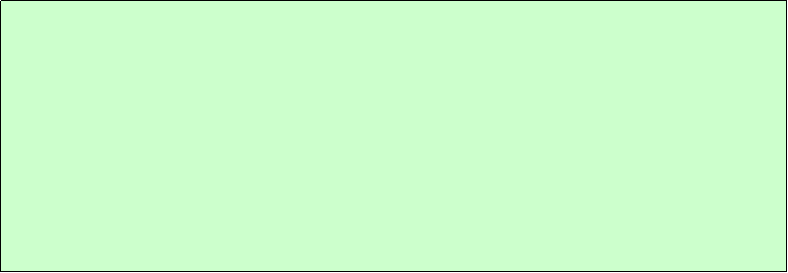A homeowner  purchased a small quantity of wood to be used in the construction of a roof  of a carport:

4 rafters each of length  6.6 metres   which cost R18.44 per metre  as well as 12 shorter roof planks, each of length 4 800 mm and which cost 90 cents less per metre than the equivalent price per metre for the 6 longer rafters.

a.       If an additional R75 transport cost is added to the cost of the wood, determine the         total cost of the planks.

b.      Determine the amount charged for VAT (VALUE ADDED TAX = 14%),  if the amount calculated includes VAT.B.     The following represent a number of floor planks of different lengths, but which are   all  of width 10 cm:

2        of length 3.3 metres;

4        of length 2.9 metres;

2    of length 2.8 metres;

1    of length 2.5 metres;

6    of length  2.4 metres;

2    of length 2.3 metres;

3    of length 2.2 metres;

3    of length 2.1 metres;

7    of length 2 metres; and

1    of length 1.3 metres

(a) How many pieces of wood (of different lengths) are there altogether?

ANS 31

(b) Determine the total length of all the floor planks altogether. ?

ANS 73.5 m

(c) Determine the maximum  area that these floor planks can cover

ANS 73.5 ×0 .10  = 7.35 square metres

 NO LENGTH PRODUCT 2 3.3 6.6 4 2.9 11.6 2 2.8 5.6 1 2.5 2.5 6 2.4 14.4 2 2.3 4.6 3 2.2 6.6 3 2.1 6.3 7 2 14 1 1.3 1.3 31 73.5In 2005 it was proposed by the educational authorities in a certain country that their teacher’s salaries would range from the equivalent of 100 000 ZAR (the South African currency) to 500 000 ZAR per annum. The salary range was divided into 75 notches, with 100 000 ZAR being the 1st notch and 500 000 ZAR being the 75th or last notch.

1          Express the ratio of 100 000 ZAR to 500 000 ZAR in its simplest form;

2          If the difference between any two consecutive notches is a constant, determine          that constant difference.

3          Is the percentage increase from one notch to the next consecutive notch also a      constant?  Motivate your answer by calculating the percentage increase from the       1st  notch to the 2nd , and that from the second last to the last notch.

SOLUTION:

1.       100 000  :  500 000      =           1  :  5

(DIVIDE BY 100 000 to reduce the ratio to its lowest terms. Remember that a ratio in its simplest form must have only whole numbers, and there must be no common factors.)

2.                 Constant Difference  =  (500 000 – 100 000)/(75-1)

=  R 5405.41

(NB: The total NUMBER of GAPS or SPACES is ONE LESS than the total NUMBER of NOTCHES.)

3.            Percentage increase from Notch 1 to Notch 2

= 5405.41/100 000*100

= 5.41%

Percentage increase from Notch 74 to Notch 75

= {[ 500 000 – (500 000 – 5405.41)]/(500 000 – 5405.41)}*100

= 1.09%

Hence the answer is clearly NO: The percentage increase becomes geometrically smaller as one progresses from Notch 1 to Notch 75.

©  DESMOND DESAI, DMD EDU-HOME, 2007

All rights reserved. Designed and created by Desmond Desai, South Africa. This page is protected by Copyright. No part of this page may be reproduced, stored in a retrieval system, or transmitted in any form of by any means, electronic, mechanical, photocopying, recording or otherwise, without the prior written permission of the copyright holder.